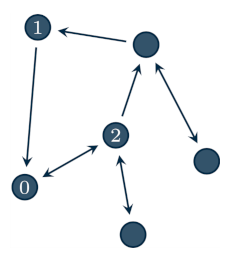# Agents in the network¶

The Agent class is meant to represent the local computing units that collaborate in the network in order to solve some specific problem.

Agents are instantiated by defining their in/out-neighbors and the weights they assign their neighbors. For example, consider the following networkThen, agent 0 is defined as:

from disropt.agents import Agent

agent = Agent(in_neighbors=[1,2],
out_neighbors=,
weights=[0.3, 0.2])


## Local data of an optimization problem¶

Assigning a local optimization problem to an agent is done via the set_problem method, which modifies the problem attribute of the agent.

Assume that the variable problem contains the local problem data, according to the procedure described in the previous page. Then, the variable is assigned to the agent by:

agent.set_problems(problem)


Local objective functions, constraints and all the operations related to the problem can be accessed through the attribute problem. For example:

agent.problem.objective_function.eval(pt) # evalate the objective function at pt
agent.problem.constraints # -> return the list of local constraints